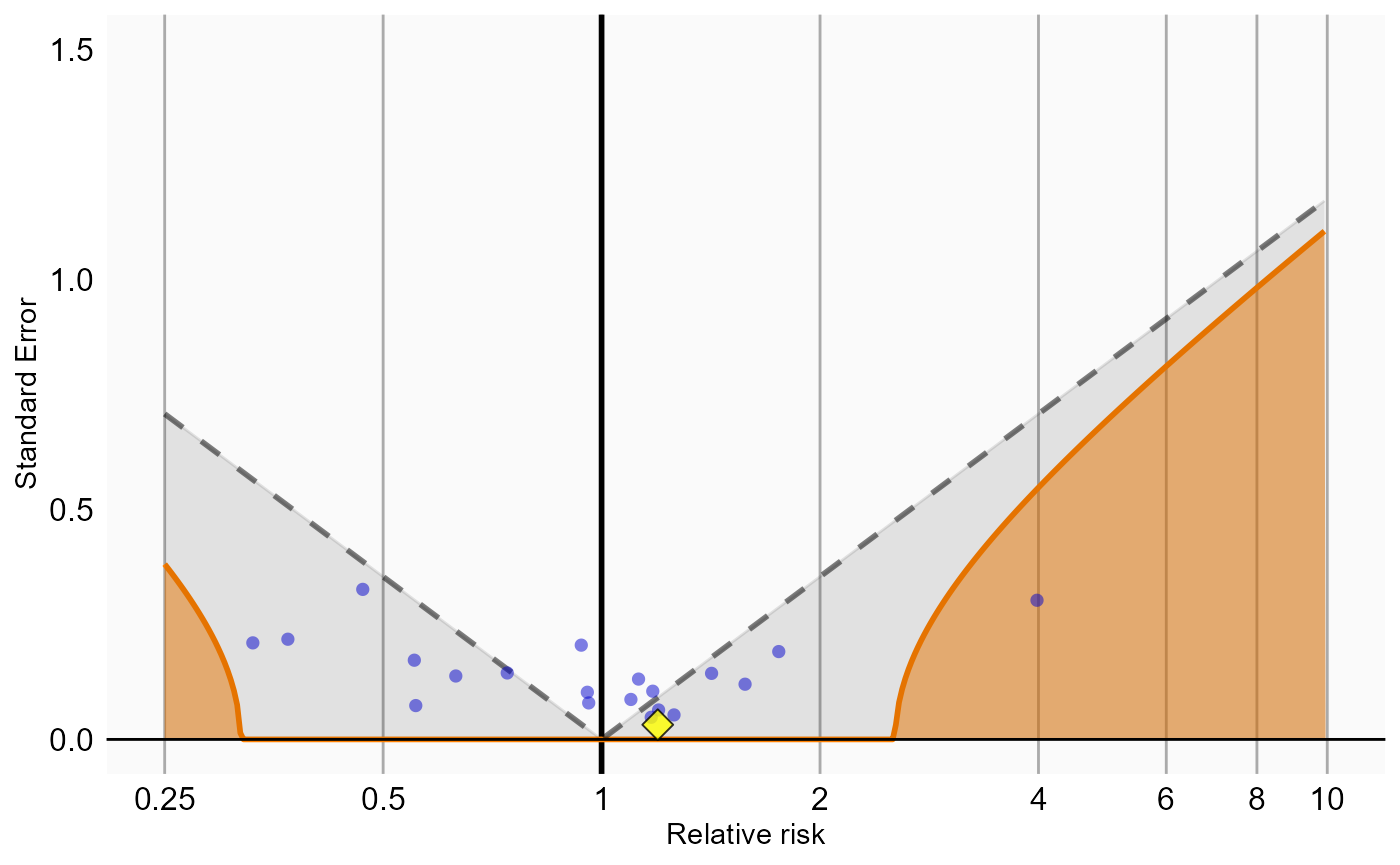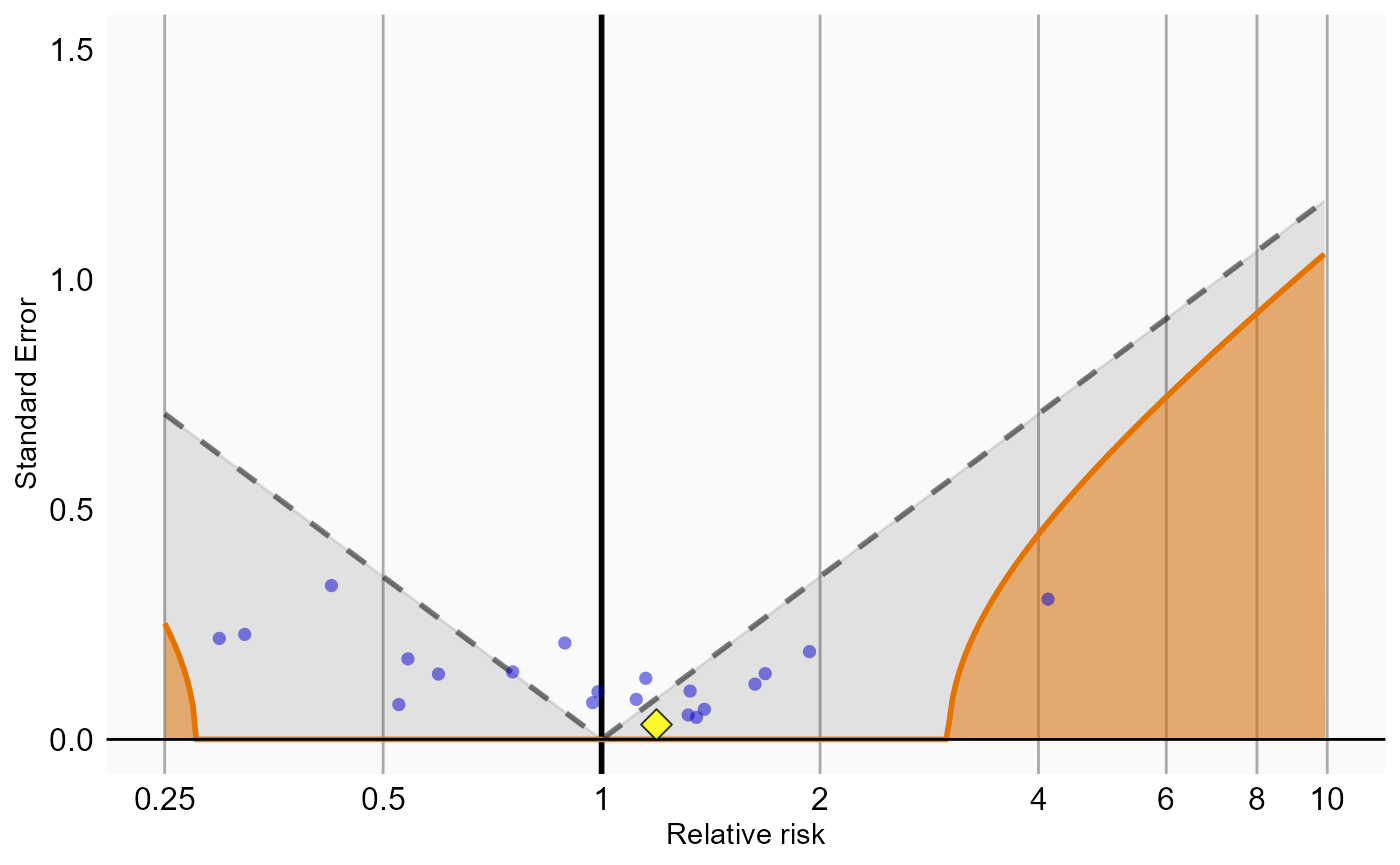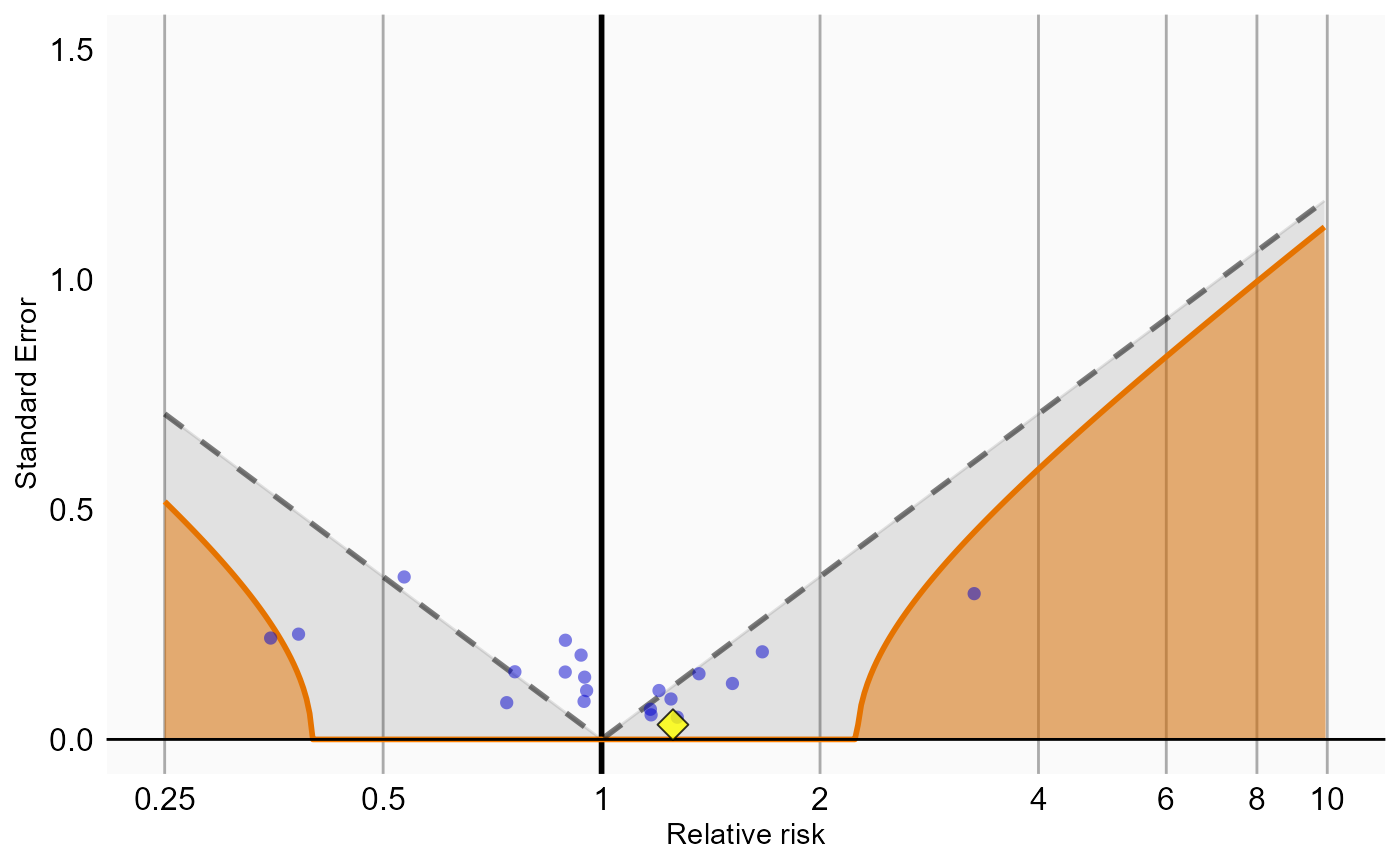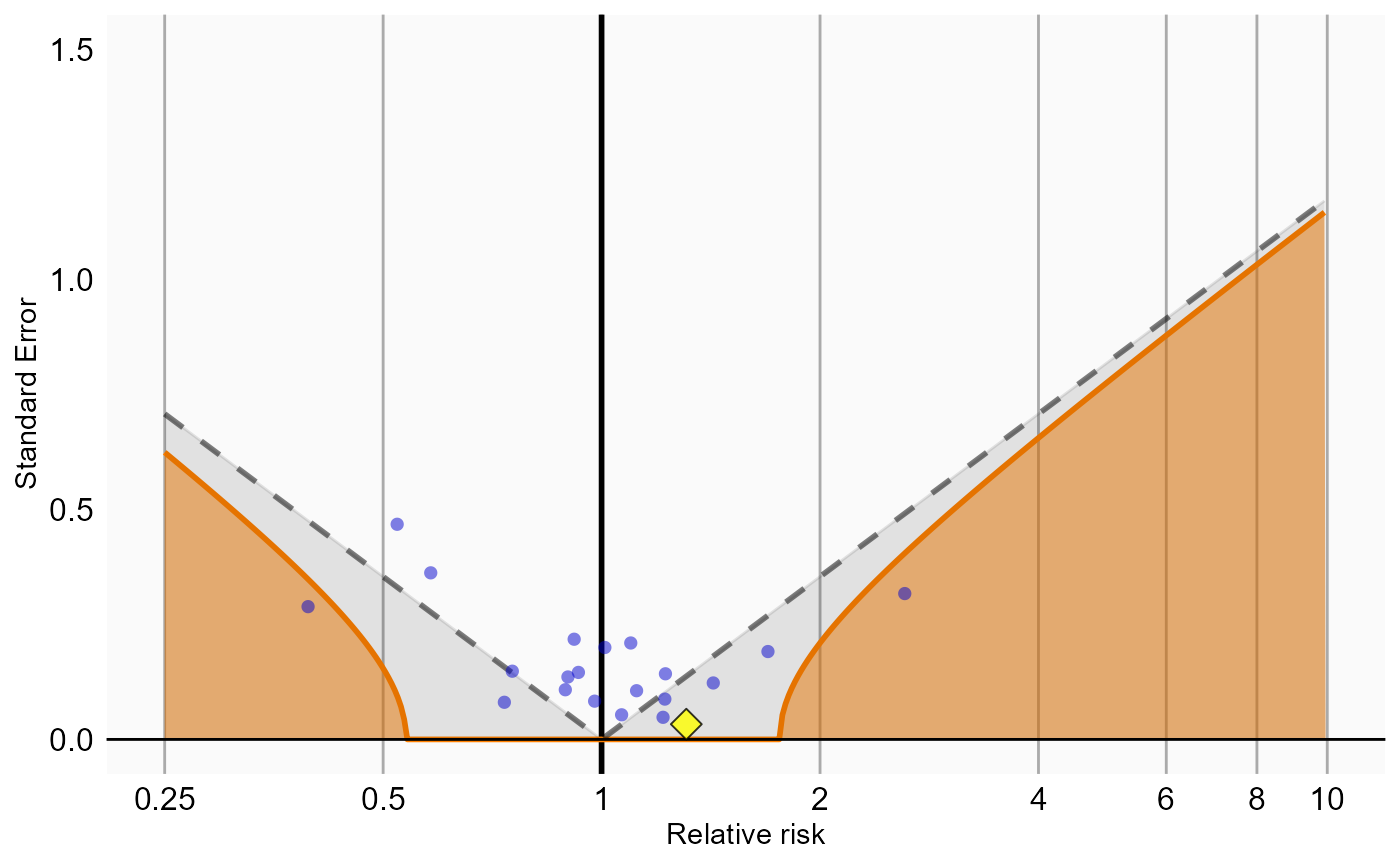# Introduction

In this vignette we focus on running several different analyses on several exposure-outcome pairs This can be useful when we want to explore the sensitivity to analyses choices, include controls, or run an experiment similar to the OMOP experiment to empirically identify the optimal analysis choices for a particular research question.

This vignette assumes you are already familiar with the SelfControlledCaseSeries package and are able to perform single studies. We will walk through all the steps needed to perform an exemplar set of analyses, and we have selected the well-studied topic of the effect of nonsteroidal anti-inflammatory drugs (NSAIDs) on gastrointestinal (GI) bleeding-related hospitalization. For simplicity, we focus on one NSAID: diclofenac. We will execute various variations of an analysis for the primary exposure pair and a large set of negative control exposures.

# General approach

The general approach to running a set of analyses is that you specify all the function arguments of the functions you would normally call, and create sets of these function arguments. The final outcome models as well as intermediate data objects will all be saved to disk for later extraction.

An analysis will be executed by calling these functions in sequence:

1. getDbSccsData()
2. createStudyPopulation()
3. createSccsIntervalData()
4. fitSccsModel()

When you provide several analyses to the SelfControlledCaseSeries package, it will determine whether any of the analyses and exposure-outcome pairs have anything in common, and will take advantage of this fact. For example, if we specify several exposure-outcome pairs with the same outcome, the data for the outcome will be extracted only once.

The function arguments you need to define have been divided into four groups:

1. Hypothesis of interest: arguments that are specific to a hypothesis of interest, in the case of the self-controlled case series this is a combination of exposure and outcome.
2. Analyses: arguments that are not directly specific to a hypothesis of interest, such as the washout window, whether to adjust for age and seasonality, etc.
3. Arguments that are the output of a previous function in the SelfControlledCaseSeries package, such as the SccsIntervalData argument of the createSccsIntervalData function. These cannot be specified by the user.
4. Arguments that are specific to an environment, such as the connection details for connecting to the server, and the name of the schema holding the CDM data.

# Preparation for the example

We need to tell R how to connect to the server where the data are. SelfControlledCaseSeries uses the DatabaseConnector package, which provides the createConnectionDetails function. Type ?createConnectionDetails for the specific settings required for the various database management systems (DBMS). For example, one might connect to a PostgreSQL database using this code:

connectionDetails <- createConnectionDetails(dbms = "postgresql",
server = "localhost/ohdsi",
user = "joe",

outputFolder <- "s:/temp/sccsVignette2"

cdmDatabaseSchema <- "my_cdm_data"
cohortDatabaseSchema <- "my_cohorts"
cdmVersion <- "5"

The last three lines define the cdmDatabaseSchema and cohortDatabaseSchema variables, as well as the CDM version. We’ll use these later to tell R where the data in CDM format live, where we want to store the (outcome) cohorts, and what version CDM is used. Note that for Microsoft SQL Server, databaseschemas need to specify both the database and the schema, so for example cdmDatabaseSchema <- "my_cdm_data.dbo".

We also need to prepare our exposures and outcomes of interest. The drug_era table in the OMOP Common Data Model already contains prespecified cohorts of users at the ingredient level, so we will use that for the exposures. For the outcomes, we want to restrict our analysis only to those events that are recorded in an inpatient setting, so we will need to create a custom cohort table. For this example, we are only interested in GI bleed (concept ID 192671) .

We create a text file called vignette.sql with the following content:

/***********************************
File vignette.sql
***********************************/

IF OBJECT_ID('@cohortDatabaseSchema.@outcomeTable', 'U') IS NOT NULL
DROP TABLE @cohortDatabaseSchema.@outcomeTable;

SELECT 1 AS cohort_definition_id,
condition_start_date AS cohort_start_date,
condition_end_date AS cohort_end_date,
condition_occurrence.person_id AS subject_id
INTO @cohortDatabaseSchema.@outcomeTable
FROM @cdmDatabaseSchema.condition_occurrence
INNER JOIN @cdmDatabaseSchema.visit_occurrence
ON condition_occurrence.visit_occurrence_id = visit_occurrence.visit_occurrence_id
WHERE condition_concept_id IN (
SELECT descendant_concept_id
FROM @cdmDatabaseSchema.concept_ancestor
WHERE ancestor_concept_id = 192671 -- GI - Gastrointestinal haemorrhage
)
AND visit_occurrence.visit_concept_id IN (9201, 9203);

Note for CDM V4 visit_concept_id should be place_of_service_concept_id, and cohort_definition_id should be cohort_concept_id.

This is parameterized SQL which can be used by the SqlRender package. We use parameterized SQL so we do not have to pre-specify the names of the CDM and result schemas. That way, if we want to run the SQL on a different schema, we only need to change the parameter values; we do not have to change the SQL code. By also making use of translation functionality in SqlRender, we can make sure the SQL code can be run in many different environments.

library(SqlRender)
sql <- render(sql,
cdmDatabaseSchema = cdmDatabaseSchema,
cohortDatabaseSchema = cohortDatabaseSchema)
result$analysisId == 1] sccsModel <- readRDS(file.path(outputFolder, sccsModelFile)) sccsModel ## SccsModel object ## ## Outcome ID: 1 ## ## Outcome count: ## outcomeSubjects outcomeEvents outcomeObsPeriods .groups ## 1 77587 177930 77741 NA ## ## Estimates: ## # A tibble: 1 x 7 ## Name ID Estimate LB95CI UB95CI logRr seLogRr ## <chr> <dbl> <dbl> <dbl> <dbl> <dbl> <dbl> ## 1 Exposure of interest: Diclofenac 1000 1.23 1.15 1.32 0.209 0.0338 Note that some of the file names will appear several times in the table. For example, all analysis share the same sccsData object. We can create a summary of the results using summarizeSccsAnalyses(): analysisSum <- summarizeSccsAnalyses(result, outputFolder) analysisSum ## # A tibble: 96 x 16 ## analysisId exposureId outcomeId outcomeSubjects outcomeEvents ## <dbl> <dbl> <dbl> <dbl> <dbl> ## 1 1 1124300 1 77587 177930 ## 2 1 705178 1 77587 177930 ## 3 1 705944 1 77587 177930 ## 4 1 710650 1 77587 177930 ## 5 1 714785 1 77587 177930 ## 6 1 719174 1 77587 177930 ## 7 1 719311 1 77587 177930 ## 8 1 735340 1 77587 177930 ## 9 1 742185 1 77587 177930 ## 10 1 780369 1 77587 177930 ## # ... with 86 more rows, and 11 more variables: outcomeObsPeriods <dbl>, ## # rr(Exposure of interest) <dbl>, ci95lb(Exposure of interest) <dbl>, ## # ci95ub(Exposure of interest) <dbl>, logRr(Exposure of interest) <dbl>, ## # seLogRr(Exposure of interest) <dbl>, rr(Pre-exposure) <dbl>, ## # ci95lb(Pre-exposure) <dbl>, ci95ub(Pre-exposure) <dbl>, ## # logRr(Pre-exposure) <dbl>, seLogRr(Pre-exposure) <dbl> This tells us, per exposure-outcome-analysis combination, the estimated relative risk and 95% confidence interval, as well as the number of subjects (cases) and the number of events observed for those subjects. ## Empirical calibration Now that we have produced estimates for all outcomes including our negative controls, we can perform empirical calibration to estimate the bias of the various analyses included in our study. We will create the calibration effect plots for every analysis ID. In each plot, the blue dots represent our negative control exposures, and the yellow diamond represents our exposure of interest: diclofenac. An unbiased, well-calibrated analysis should have 95% of the negative controls between the dashed lines (ie. 95% should have p > .05). install.packages("EmpiricalCalibration") library(EmpiricalCalibration) # Analysis 1: Simplest model negCons <- analysisSum[analysisSum$analysisId == 1 & analysisSum$exposureId != 1124300, ] ei <- analysisSum[analysisSum$analysisId == 1 & analysisSum$exposureId == 1124300, ] null <- fitNull(negCons$logRr(Exposure of interest),
negCons$seLogRr(Exposure of interest)) plotCalibrationEffect(logRrNegatives = negCons$logRr(Exposure of interest),
seLogRrNegatives = negCons$seLogRr(Exposure of interest), logRrPositives = ei$logRr(Exposure of interest),
seLogRrPositives = ei$seLogRr(Exposure of interest), null) ## Warning in fitNull(negCons$logRr(Exposure of interest),
## negCons$seLogRr(Exposure of interest)): Estimate(s) with NA standard error ## detected. Removing before fitting null distribution ## Warning: Removed 2 rows containing missing values (geom_point).# Analysis 2: Including prophylactics negCons <- analysisSum[analysisSum$analysisId == 2 & analysisSum$exposureId != 1124300, ] ei <- analysisSum[analysisSum$analysisId == 2 & analysisSum$exposureId == 1124300, ] null <- fitNull(negCons$logRr(Exposure of interest),
negCons$seLogRr(Exposure of interest)) plotCalibrationEffect(logRrNegatives = negCons$logRr(Exposure of interest),
seLogRrNegatives = negCons$seLogRr(Exposure of interest), logRrPositives = ei$logRr(Exposure of interest),
seLogRrPositives = ei$seLogRr(Exposure of interest), null) ## Warning in fitNull(negCons$logRr(Exposure of interest),
## negCons$seLogRr(Exposure of interest)): Estimate(s) with NA standard error ## detected. Removing before fitting null distribution ## Warning: Removed 2 rows containing missing values (geom_point).# Analysis 3: Including prophylactics, age, season, pre-exposure, and censoring negCons <- analysisSum[analysisSum$analysisId == 3 & analysisSum$exposureId != 1124300, ] ei <- analysisSum[analysisSum$analysisId == 3 & analysisSum$exposureId == 1124300, ] null <- fitNull(negCons$logRr(Exposure of interest),
negCons$seLogRr(Exposure of interest)) plotCalibrationEffect(logRrNegatives = negCons$logRr(Exposure of interest),
seLogRrNegatives = negCons$seLogRr(Exposure of interest), logRrPositives = ei$logRr(Exposure of interest),
seLogRrPositives = ei$seLogRr(Exposure of interest), null) ## Warning in fitNull(negCons$logRr(Exposure of interest),
## negCons$seLogRr(Exposure of interest)): Estimate(s) with NA standard error ## detected. Removing before fitting null distribution ## Warning: Removed 2 rows containing missing values (geom_point).# Analysis 4: Including all other drugs (as well as prophylactics, age, season, pre- # exposure, and censoring) negCons <- analysisSum[analysisSum$analysisId == 4 & analysisSum$exposureId != 1124300, ] ei <- analysisSum[analysisSum$analysisId == 4 & analysisSum$exposureId == 1124300, ] null <- fitNull(negCons$logRr(Exposure of interest),
negCons$seLogRr(Exposure of interest)) plotCalibrationEffect(logRrNegatives = negCons$logRr(Exposure of interest),
seLogRrNegatives = negCons$seLogRr(Exposure of interest), logRrPositives = ei$logRr(Exposure of interest),
seLogRrPositives = ei$seLogRr(Exposure of interest), null) ## Warning in fitNull(negCons$logRr(Exposure of interest),
## negCons\$seLogRr(Exposure of interest)): Estimate(s) with NA standard error
## detected. Removing before fitting null distribution
## Warning: Removed 2 rows containing missing values (geom_point).# Acknowledgments

Considerable work has been dedicated to provide the SelfControlledCaseSeries package.

citation("SelfControlledCaseSeries")
##
## To cite package 'SelfControlledCaseSeries' in publications use:
##
##   Martijn Schuemie, Patrick Ryan, Trevor Shaddox and Marc Suchard
##   (2020). SelfControlledCaseSeries: Self-Controlled Case Series. R
##   package version 2.0.0.
##   https://github.com/OHDSI/SelfControlledCaseSeries
##
## A BibTeX entry for LaTeX users is
##
##   @Manual{,
##     title = {SelfControlledCaseSeries: Self-Controlled Case Series},
##     author = {Martijn Schuemie and Patrick Ryan and Trevor Shaddox and Marc Suchard},
##     year = {2020},
##     note = {R package version 2.0.0},
##     url = {https://github.com/OHDSI/SelfControlledCaseSeries},
##   }

Further, SelfControlledCaseSeries makes extensive use of the Cyclops package.

citation("Cyclops")
##
## To cite Cyclops in publications use:
##
## Suchard MA, Simpson SE, Zorych I, Ryan P, Madigan D (2013). "Massive
## parallelization of serial inference algorithms for complex generalized
## linear models." _ACM Transactions on Modeling and Computer Simulation_,
## *23*, 10. <URL: http://dl.acm.org/citation.cfm?id=2414791>.
##
## A BibTeX entry for LaTeX users is
##
##   @Article{,
##     author = {M. A. Suchard and S. E. Simpson and I. Zorych and P. Ryan and D. Madigan},
##     title = {Massive parallelization of serial inference algorithms for complex generalized linear models},
##     journal = {ACM Transactions on Modeling and Computer Simulation},
##     volume = {23},
##     pages = {10},
##     year = {2013},
##     url = {http://dl.acm.org/citation.cfm?id=2414791},
##   }

This work is supported in part through the National Science Foundation grant IIS 1251151.